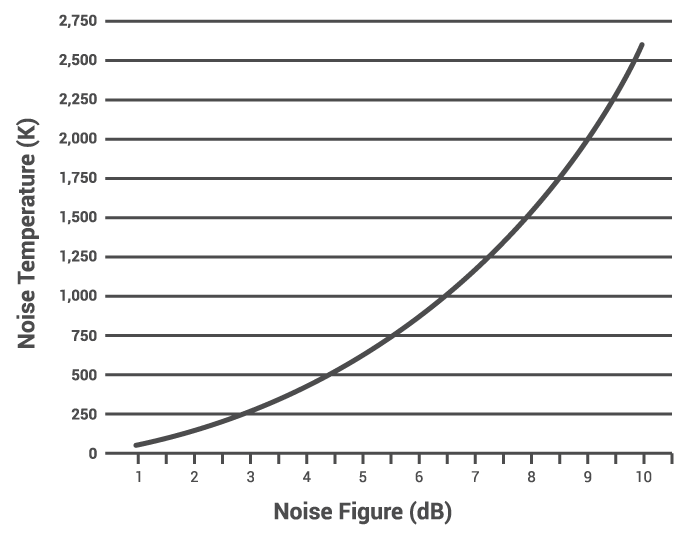# Noise Figure and Noise Temperature Calculator

## This calculator computes the noise figure and noise temperature in a system

(K)

### Overview

An electronic system's noise figure is the ratio of the signal-to-noise at the input to that of the output. It is a useful parameter to determine how much the signal is reduced at the output because of the presence of noise. Meanwhile, a system's noise temperature is a function of the total noise power in the circuit, and the bandwidth of frequencies involved. The noise figure and noise temperature are related and can be computed using the formulas below. This tool helps you compute the noise figure of a system given the noise temperature, or the noise temperature given the noise figure.### Equations

$$NF = 10log_{10}\left[\frac{T_{noise}}{T_{ref}}+1\right]$$

Where:

$$NF$$ = noise figure

$$T_{noise}$$ = noise temperature

$$T_{ref}$$ = reference temperature. Normally the ambient temperature.

### Applications

In an electronic communications system, the transmitted signal will always be attenuated and corrupted with additive noise, which often results in a very weak signal at the receiver. Noise in the receiver can come from thermal noise or from "processes" that generate noise. These processes will have a white spectrum,  are are typically identical to thermal noise. Since they are indistinguishable, the contributions of all noise sources can be lumped together and regarded as a level of thermal noise. The noise power spectral density generated by all of these sources can be described by assigning to the noise a temperature (T). The noise figure of the system describes the effect of the noise temperature to the overall noise of the system. Looking at the formula above, if the thermal noise is zero, the noise figure is also zero. Note that there is no electronic system that will have a noise temperature of zero, which means the noise figure can also never be zero.